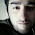## Wednesday, January 7, 2015

### Cyclic Process,Reversible Process and Work Done Graphs

Equation of state is the relation among the physical quantities which are fundamental physical quantities in thermodynamics. They are nothing but the relation among the coordinates of thermodynamics.

The locus of series of the points representing the states through which the system passes is called as a path.

The complete description of the change of the state along which the path that taken is called process.

Quasi static process is a very small and slow process in which system goes to different states.

The process in which system after passing through various states of pressure, volume and temperature changes, which returns to its initial state is called as a cyclic process.

Problem and solution

In any cyclic process, the pressure and volume  graph is the closed curve. Work done in this process is calibrated as the area of the graph.

Reversible process

The process that can be retraced back in the opposite direction in such a way that the system passes to the same states and finally the system and surroundings returns to their original states. This shall be a very slow process.

A process that cannot be retraced back in opposite direction is called as a irreversible process.

Graphical representation of the work using PV diagrams

To calculate the work done we shall try a graph taking the change in volume and x-axis and the change in pressure on y-axis.

When the pressure is constant and the graph is just straight line parallel to x-axis.
When the process is a cyclic process the graph is a closed curve as shown below.

And some more graphs are as shown below.

The process in which the pressure of a system is always kept constant is called as a isobaric process. In this process to calculate the work done we need not go for the process of integration.

The process in which the volume of a system is kept constant is called as isochoric process. In this process as the volume is kept constant the work done is equal to 0. All the heat energy supplied is equal to only change in internal energy.

In a cyclic process the change in internal energy of the system is equal to 0. It means the initial and final internal energies of the system are equal.

The slope of a adiabatic graph between the pressure and volume will be more than that of the isothermal process.

Related Posts

#### 1 comment:

1.